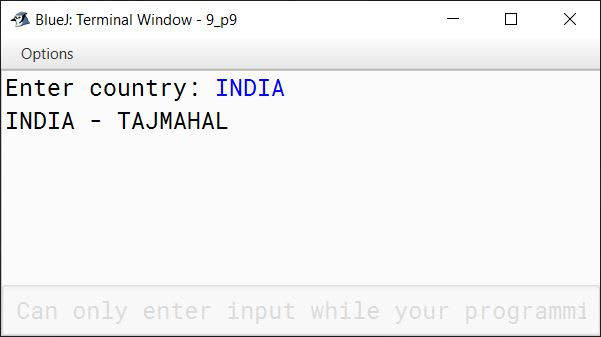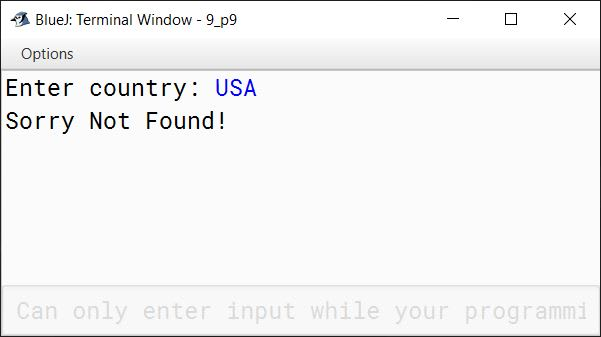# Solved 2016 Question Paper ICSE Class 10 Computer Applications

## Section A

#### Question 1

(a) Define Encapsulation.

Encapsulation is a mechanism that binds together code and the data it manipulates. It keeps them both safe from the outside world, preventing any unauthorised access or misuse. Only member methods, which are wrapped inside the class, can access the data and other methods.

(b) What are keywords ? Give an example.

Keywords are reserved words that have a special meaning to the Java compiler. Example: class, public, int, etc.

(c) Name any two library packages.

Two library packages are:

1. java.io
2. java.util

(d) Name the type of error ( syntax, runtime or logical error) in each case given below:

1. Math.sqrt (36 – 45)
2. int a;b;c;

1. Runtime Error
2. Syntax Error

(e) If int x[] = { 4, 3, 7, 8, 9, 10}; what are the values of p and q ?

1. p = x.length
2. q = x + x * x

1. 6
2. q = x + x * x
q = 7 + 10 * 3
q = 7 + 30
q = 37

#### Question 2

(a) State the difference between == operator and equals() method.

equals()==
It is a methodIt is a relational operator
It is used to check if the contents of two strings are same or notIt is used to check if two variables refer to the same object in memory
Example:
String s1 = new String("hello");
String s2 = new String("hello");
boolean res = s1.equals(s2);
System.out.println(res);

The output of this code snippet is true as contents of s1 and s2 are the same.
Example:
String s1 = new String("hello");
String s2 = new String("hello");
boolean res = s1 == s2;
System.out.println(res);

The output of this code snippet is false as s1 and s2 point to different String objects.

(b) What are the types of casting shown by the following examples:

1. char c = (char) 120;
2. int x = ‘t’;

1. Explicit Cast.
2. Implicit Cast.

(c) Differentiate between formal parameter and actual parameter.

Formal parameterActual parameter
Formal parameters appear in function definition.Actual parameters appear in function call statement.
They represent the values received by the called function.They represent the values passed to the called function.

(d) Write a function prototype of the following:
A function PosChar which takes a string argument and a character argument and returns an integer value.

int PosChar(String s, char ch)

(e) Name any two types of access specifiers.

1. public
2. private

#### Question 3

(a) Give the output of the following string functions:

1. "MISSISSIPPI".indexOf('S') + "MISSISSIPPI".lastIndexOf('I')

1. 2 + 10 = 12
2. ASCII Code of B - ASCII Code of D
⇒ 66 - 68
⇒ -2

(b) Give the output of the following Math functions:

1. Math.ceil(4.2)
2. Math.abs(-4)

1. 5.0
2. 4

(c) What is a parameterized constructor ?

A Parameterised constructor receives parameters at the time of creating an object and initializes the object with the received values.

(d) Write down java expression for:

$T = \sqrt{A^2 + B^2 + C^2}$

T = Math.sqrt(a*a + b*b + c*c)

(e) Rewrite the following using ternary operator:

if (x%2 == 0)
System.out.print("EVEN");
else
System.out.print("ODD");

System.out.print(x%2 == 0 ? "EVEN" : "ODD");

(f) Convert the following while loop to the corresponding for loop:

int m = 5, n = 10;
while (n>=1)
{
System.out.println(m*n);
n--;
}

int m = 5;
for (int n = 10; n >= 1; n--) {
System.out.println(m*n);
}

(g) Write one difference between primitive data types and composite data types.

Primitive Data Types are built-in data types defined by Java language specification whereas Composite Data Types are defined by the programmer.

(h) Analyze the given program segment and answer the following questions:

1. Write the output of the program segment.
2. How many times does the body of the loop gets executed ?
for(int m = 5; m <= 20; m += 5)
{    if(m % 3 == 0)
break;
else
if(m % 5 == 0)
System.out.println(m);
continue;
}

##### Output
5
10


Loop executes 3 times.

Below dry run of the loop explains its working.

mOutputRemarks
551st Iteration
105
10
2nd Iteration
155
10
3rd Iteration — As m % 3 becomes true, break statement exists the loop.

(i) Give the output of the following expression:

a+= a++ + ++a + --a + a-- ; when a = 7

a+= a++ + ++a + --a + a--
⇒ a = a + (a++ + ++a + --a + a--)
⇒ a = 7 + (7 + 9 + 8 + 8)
⇒ a = 7 + 32
⇒ a = 39

(j) Write the return type of the following library functions:

1. isLetterOrDigit(char)
2. replace(char, char)

1. boolean
2. String

## Section B

#### Question 4

Define a class named BookFair with the following description:

Instance variables/Data members:
String Bname — stores the name of the book
double price — stores the price of the book

Member methods:
(i) BookFair() — Default constructor to initialize data members
(ii) void Input() — To input and store the name and the price of the book.
(iii) void calculate() — To calculate the price after discount. Discount is calculated based on the following criteria.

PriceDiscount
Less than or equal to ₹10002% of price
More than ₹1000 and less than or equal to ₹300010% of price
More than ₹300015% of price

(iv) void display() — To display the name and price of the book after discount.

Write a main method to create an object of the class and call the above member methods.

import java.util.Scanner;

public class BookFair
{
private String bname;
private double price;

public BookFair() {
bname = "";
price = 0.0;
}

public void input() {
Scanner in = new Scanner(System.in);
System.out.print("Enter name of the book: ");
bname = in.nextLine();
System.out.print("Enter price of the book: ");
price = in.nextDouble();
}

public void calculate() {
double disc;
if (price <= 1000)
disc = price * 0.02;
else if (price <= 3000)
disc = price * 0.1;
else
disc = price * 0.15;

price -= disc;
}

public void display() {
System.out.println("Book Name: " + bname);
System.out.println("Price after discount: " + price);
}

public static void main(String args[]) {
BookFair obj = new BookFair();
obj.input();
obj.calculate();
obj.display();
}
}
##### Output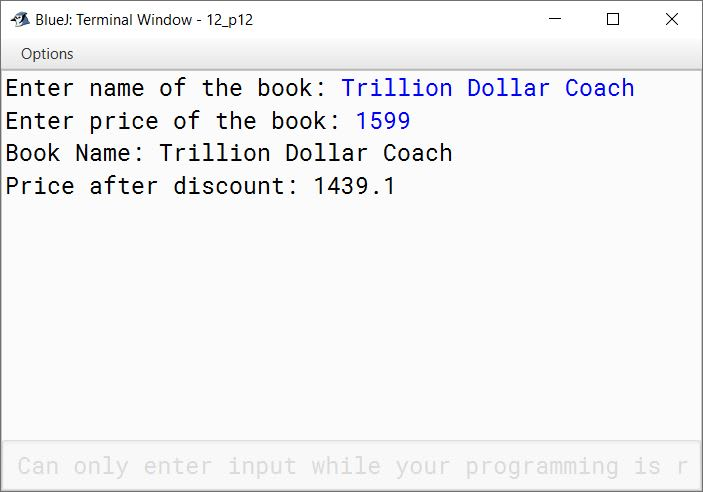#### Question 5

Using the switch statement, write a menu driven program for the following:

(i) To print the Floyd’s triangle [Given below]

1
2   3
4   5   6
7   8   9  10
11 12 13 14 15

(b) To display the following pattern:
I
I C
I C S
I C S E

For an incorrect option, an appropriate error message should be displayed.

import java.util.Scanner;

public class KboatPattern
{
public static void main(String args[]) {
Scanner in = new Scanner(System.in);
System.out.println("Type 1 for Floyd's triangle");
System.out.println("Type 2 for an ICSE pattern");

int ch = in.nextInt();

switch (ch) {
case 1:
int a = 1;
for (int i = 1; i <= 5; i++) {
for (int j = 1; j <= i; j++) {
System.out.print(a++ + "\t");
}
System.out.println();
}
break;

case 2:
String s = "ICSE";
for (int i = 0; i < s.length(); i++) {
for (int j = 0; j <= i; j++) {
System.out.print(s.charAt(j) + " ");
}
System.out.println();
}
break;

default:
System.out.println("Incorrect Choice");
}
}
}
##### Output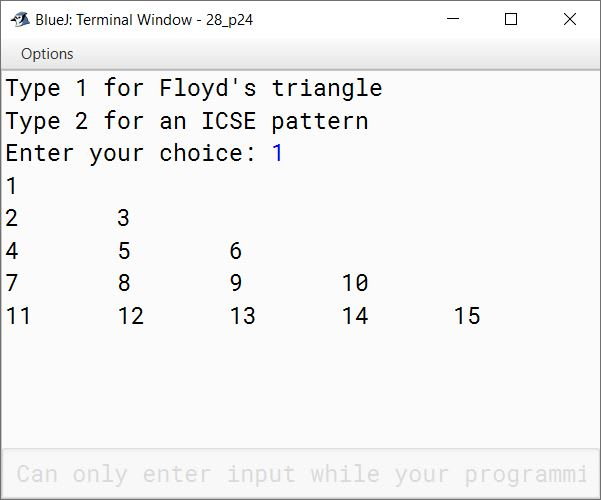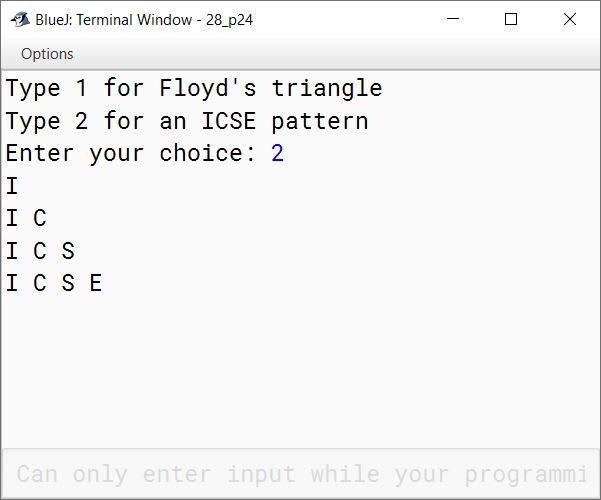#### Question 6

Special words are those words which start and end with the same letter.
Example: EXISTENCE, COMIC, WINDOW
Palindrome words are those words which read the same from left to right and vice-versa.
Example: MALYALAM, MADAM, LEVEL, ROTATOR, CIVIC
All palindromes are special words but all special words are not palindromes.

Write a program to accept a word. Check and display whether the word is a palindrome or only a special word or none of them.

import java.util.Scanner;

public class KboatSpecialPalindrome
{
public static void main(String args[]) {
Scanner in = new Scanner(System.in);
System.out.print("Enter a word: ");
String str = in.next();
str = str.toUpperCase();
int len = str.length();

if (str.charAt(0) == str.charAt(len - 1)) {
boolean isPalin = true;
for (int i = 1; i < len / 2; i++) {
if (str.charAt(i) != str.charAt(len - 1 - i)) {
isPalin = false;
break;
}
}

if (isPalin) {
System.out.println("Palindrome");
}
else {
System.out.println("Special");
}
}
else {
System.out.println("Neither Special nor Palindrome");
}

}
}
##### Output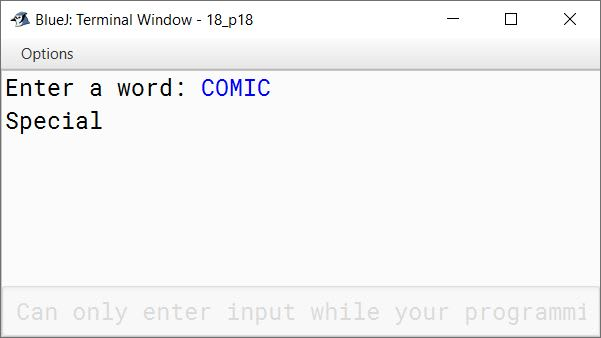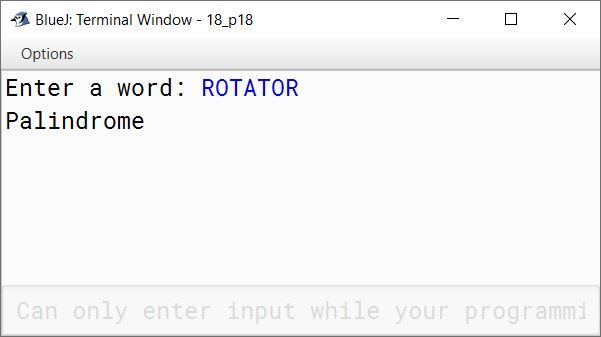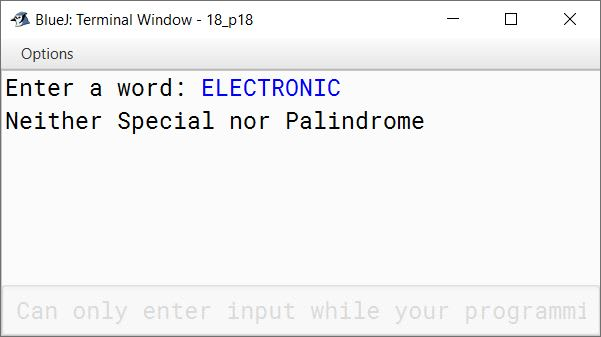#### Question 7

Design a class to overload a function sumSeries() as follows:

(i) void sumSeries(int n, double x): with one integer argument and one double argument to find and display the sum of the series given below:

$s = \dfrac{x}{1} - \dfrac{x}{2} + \dfrac{x}{3} - \dfrac{x}{4} + \dfrac{x}{5} ...\space ...\space ...\space to \space n \space terms$

(ii) void sumSeries(): to find and display the sum of the following series:

$s = 1 + (1 \times 2) + (1 \times 2 \times 3) + ...\space ...\space ...\space + (1 \times 2 \times 3 \times 4 ...\space ...\space ...\space \times 20)$

public class KboatOverload
{
void sumSeries(int n, double x) {
double sum = 0;
for (int i = 1; i <= n; i++) {
double t = x / i;
if (i % 2 == 0)
sum -= t;
else
sum += t;
}
System.out.println("Sum = " + sum);
}

void sumSeries() {
long sum = 0, term = 1;
for (int i = 1; i <= 20; i++) {
term *= i;
sum += term;
}
System.out.println("Sum=" + sum);
}
}
##### Output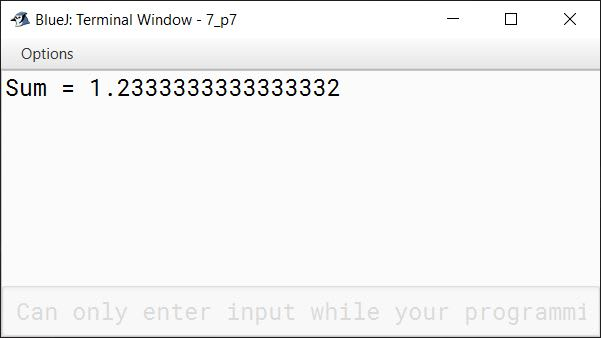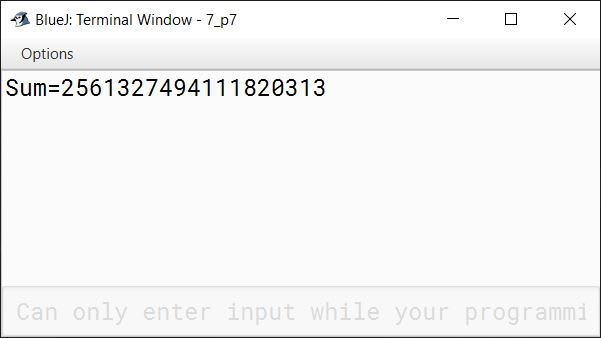#### Question 8

Write a program to accept a number and check and display whether it is a Niven number or not.
(Niven number is that number which is divisible by its sum of digits.).

Example:
Consider the number 126. Sum of its digits is 1 + 2 + 6 = 9 and 126 is divisible by 9.

import java.util.Scanner;

public class KboatNivenNumber
{
public void checkNiven() {
Scanner in = new Scanner(System.in);
System.out.print("Enter number: ");
int num = in.nextInt();
int orgNum = num;

int digitSum = 0;

while (num != 0) {
int digit = num % 10;
num /= 10;
digitSum += digit;
}

/*
* digitSum != 0 check prevents
* division by zero error for the
* case when users gives the number
* 0 as input
*/
if (digitSum != 0 && orgNum % digitSum == 0)
System.out.println(orgNum + " is a Niven number");
else
System.out.println(orgNum + " is not a Niven number");
}
}
##### Output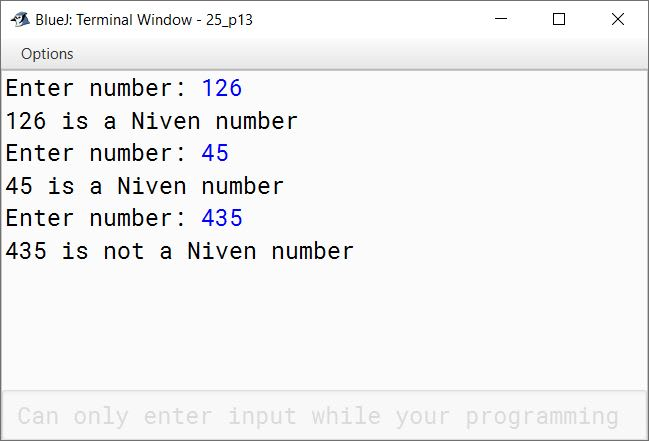#### Question 9

Write a program to initialize the seven Wonders of the World along with their locations in two different arrays. Search for a name of the country input by the user. If found, display the name of the country along with its Wonder, otherwise display "Sorry not found!".

Seven Wonders:
CHICHEN ITZA, CHRIST THE REDEEMER, TAJ MAHAL, GREAT WALL OF CHINA, MACHU PICCHU, PETRA, COLOSSEUM

Locations:
MEXICO, BRAZIL, INDIA, CHINA, PERU, JORDAN, ITALY

Examples:
Country name: INDIA
Output: TAJ MAHAL

Country name: USA

import java.util.Scanner;

public class Kboat7Wonders
{
public static void main(String args[]) {

String wonders[] = {"CHICHEN ITZA", "CHRIST THE REDEEMER", "TAJMAHAL",
"GREAT WALL OF CHINA", "MACHU PICCHU", "PETRA", "COLOSSEUM"};

String locations[] = {"MEXICO", "BRAZIL", "INDIA", "CHINA", "PERU", "JORDAN",
"ITALY"};

Scanner in = new Scanner(System.in);
System.out.print("Enter country: ");
String c = in.nextLine();
int i;

for (i = 0; i < locations.length; i++) {
if (locations[i].equals(c)) {
System.out.println(locations[i] + " - " + wonders[i]);
break;
}
}

if (i == locations.length)
}